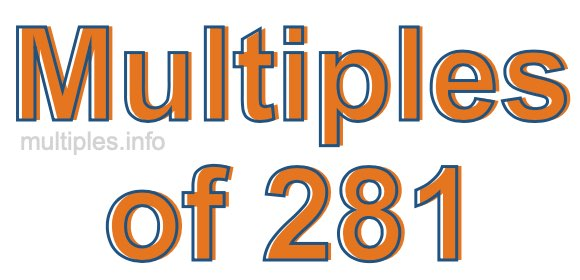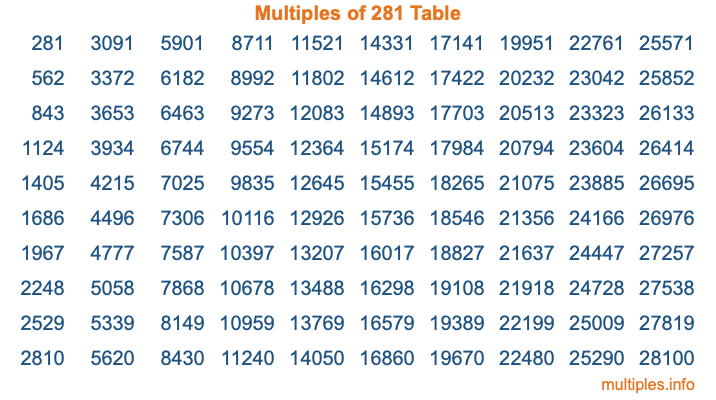Multiples of 281Welcome to the Multiples of 281 page. Here we will first teach you everything you will ever need to know about the multiples of 281, and then give you a study guide summary of everything we taught you to make sure you remember it all. Use this page to look up facts and learn information about the multiples of 281. This page will make you a multiples of two hundred eighty-one expert!

Definition of Multiples of 281
Multiples of 281 are all the numbers that when divided by 281 equal an integer. Each of the multiples of 281 are called a multiple. A multiple of 281 is created by multiplying 281 by an integer.

Therefore, to create a list of multiples of 281, you start with 1 multiplied by 281, then 2 multiplied by 281, then 3 multiplied by 281, and so on for as long as you want. Thus, the list of the first five multiples of 281 is 281, 562, 843, 1124, and 1405. To see a larger list of multiples of 281, see the printable image of Multiples of 281 further down on this page. We also have a category where you can choose any nth multiple of 281.

Multiples of 281 Checker
The Multiples of 281 Checker below checks to see if any number of your choice is a multiple of 281. In other words, it checks to see if there is any number (integer) that when multiplied by 281 will equal your number. To do that, we divide your number by 281. If the the quotient is an integer, then your number is a multiple of 281.

Is  a multiple of 281?

Least Common Multiple of 281 and ...
A Least Common Multiple (LCM) is the lowest multiple that two or more numbers have in common. This is also called the smallest common multiple or lowest common multiple and is useful to know when you are adding our subtracting fractions. Enter one or more numbers below (281 is already entered) to find the LCM.

Check out our LCM Calculator if you need more details about the Least Common Multiple or if you need the LCM for different numbers for adding and subtraction fractions.

nth Multiple of 281
As we stated above, 281 is the first multiple of 281, 562 is the second multiple of 281, 843 is the third multiple of 281, and so on. Enter a number below to find the nth multiple of 281.

th multiple of 281

Multiples of 281 vs Factors of 281
281 is a multiple of 281 and a factor of 281, but that is where the similarities end. All postive multiples of 281 are 281 or greater than 281. All positive factors of 281 are 281 or less than 281.

Below is the beginning list of multiples of 281 and the factors of 281 so you can compare:

Multiples of 281: 281, 562, 843, 1124, 1405, etc.

Factors of 281: 1, 281

As you can see, the multiples of 281 are all the numbers that you can divide by 281 to get a whole number. The factors of 281, on the other hand, are all the whole numbers that you can multiply by another whole number to get 281.

It's also interesting to note that if a number (x) is a factor of 281, then 281 will also be a multiple of that number (x).

Multiples of 281 vs Divisors of 281
The divisors of 281 are all the integers that 281 can be divided by evenly. Below is a list of the divisors of 281.

Divisors of 281: 1, 281

The interesting thing to note here is that if you take any multiple of 281 and divide it by a divisor of 281, you will see that the quotient is an integer.

Multiples of 281 Table
Below is an image of the first 100 multiples of 281 in a table. The table is in chronological order, column by column. The first column has the first ten multiples of 281, the second column has the next ten multiples of 281, and so on.The Multiples of 281 Table is also referred to as the 281 Times Table or Times Table of 281. You are welcome to print out our table for your studies.

Negative Multiples of 281
Although not often discussed or needed in math, it is worth mentioning that you can make a list of negative multiples of 281 by multiplying 281 by -1, then by -2, then by -3, and so on, to get the following list of negative multiples of 281:

-281, -562, -843, -1124, -1405, etc.

Multiples of 281 Summary
Below is a summary of important Multiples of 281 facts that we have discussed on this page. To retain the knowledge on this page, we recommend that you read through the summary and explain to yourself or a study partner why they hold true.

There are an infinite number of multiples of 281.

A multiple of 281 divided by 281 will equal a whole number.

281 divided by a factor of 281 equals a divisor of 281.

The nth multiple of 281 is n times 281.

The largest factor of 281 is equal to the first positive multiple of 281.

281 is a multiple of every factor of 281.

281 is a multiple of 281.

A multiple of 281 divided by a divisor of 281 equals an integer.

281 divided by a divisor of 281 equals a factor of 281.

Any integer times 281 will equal a multiple of 281.

Multiples of a Number
Here you can get the multiples of another number, all with the same attention to detail as we did for multiples of 281 on this page.

Multiples of
Multiples of 282
Did you find our page about multiples of two hundred eighty-one educational? Do you want more knowledge? Check out the multiples of the next number on our list!